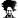# How to alter VBA code

#### Jet Fusion

##### Member
Hi all

Please can someone assit in changing the below code to not include the hyperlinks but still automatically create an index page of all the tabs in a workbook.

Code:
``````Private Sub Worksheet_Activate()
Dim wSheet As Worksheet
Dim M As Long
M = 1
With Me
.Columns(1).ClearContents
.Cells(1, 1) = "INDEX"
.Cells(1, 1).Name = "Index"
End With

For Each wSheet In Worksheets
If wSheet.Name <> Me.Name Then
M = M + 1
With wSheet
.Range("H1").Name = "Start" & wSheet.Index
.Hyperlinks.Add Anchor:=.Range("H1"), Address:="", SubAddress:="Index", TextToDisplay:="Back to Index"
End With
Me.Hyperlinks.Add Anchor:=Me.Cells(M, 1), Address:="", SubAddress:="Start" & wSheet.Index, TextToDisplay:=wSheet.Name
End If
Next wSheet
End Sub``````
Thank you#### Hui

##### Excel Ninja
Staff member
Try this:

Code:
``````Private Sub Worksheet_Activate()
Dim wSheet As Worksheet
Dim M As Long
M = 1
With Me
.Columns(1).ClearContents
.Cells(1, 1) = "INDEX"
.Cells(1, 1).Name = "Index"
End With

For Each wSheet In Worksheets
If wSheet.Name <> Me.Name Then
M = M + 1
Me.Cells(M, 1) = wSheet.Name
End If
Next wSheet
End Sub``````
Save it in the worksheet module where you want the index
eg:•Jet Fusion

#### Jet Fusion

##### Member
Hi @Hui

Thank you the code worked